# LM13700 as VCR wrong values

#### jacopo1919

Joined Apr 12, 2020
80
Hello. I'm having trouble to figure out why my calculations don't match the measurements when I hook up a LM13700 as Voltage controlled resistor.
Similar issue for both configurations: single ended or floating.

as for LM13700 Datasheet (fig.28, Floating Voltage-Controlled Resistor)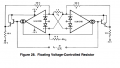THEORY:
the formula given is Rx= (2 * R)/(gm * RA) , where gm is 19.2 * Ibias
In my calculations,
Ibias is max 0.42 mA
Ra is 1K
R is 100K
The Rx resulting should be 24.8 K and for descreasing Ibias, Rx goes up.

following the circuit , i feed max 0.42 mA at Pin 1 and 16 of the LM13700 and i set up a simple voltage divider to test it in practice as follows.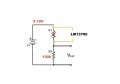Vin is 5.19 V, R1 is replaced with the LM13700 and r2 is 100k.

Following the theoric calculations, i should expect Vout =4.16.

But what i can measure in practice is 3.36V though.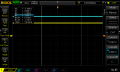Do you have any suspects or hints to give?

I take for granted that Ibias of 0.42 mA that i split between pin 1 and 16 of the OTA, has to be used as it is in the Formula ( and so i don't have to use 0.42mA/2)

Thanks a lot for any advice or help.

Last edited:

#### jacopo1919

Joined Apr 12, 2020
80
First time i use LTspice so excuse me if I mistake something.
This should be easier to understand than my monologue above.
I attach the files for this simulation if you are interested (i guess they are enough to run it).

It looks to me is that my practical measurments match the LTspice simulation since i get 3.2V at the output.
So, I'm wondering what I'm doing wrong in my theorical calculations.

I'm missing a very stupid point somewhere but I can't figure it out.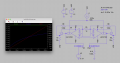#### Attachments

• 5.6 KB Views: 3
• 1.7 KB Views: 5
• 1.4 KB Views: 4

#### ericgibbs

Joined Jan 29, 2010
15,544
hi,
formula given is Rx= (2 * R)/(gm * RA) , where gm is 19.2 * Ibias

gm= 19.2*0.42 =8.064

Rx= 200k/(8.064*1k) =24.8k

Vo = 5.19v *( 100/128.4) 5.19v * 0.7788 = 4.042Vo

The LTS sim shows ~+3v.

E
Corrected typo.

Last edited:
•jacopo1919

#### ericgibbs

Joined Jan 29, 2010
15,544

#### Attachments

• 2.8 KB Views: 5

#### jacopo1919

Joined Apr 12, 2020
80
Datasheet says :**The Bias Input pins (pins 1 or 16), are 2 diode drops above the negative supply, and therefore
VBIAS = 2(VBE) + V- , which for this application is -13.6 V. To set IBIAS to 1ma when VC = 15 V requires a 28.6-kΩ;
**,
this because 15V -(-13.6V)/28.6k=1mA

so in our case VBIAS= 2*(0.7) + (-12V) = -10.6V.

In your simulation, VC sweeps from 0 to 6V through 15k (R1).
So the relative current Iabc sweeps from 0V -(-10.6V)/15k=0.7mA to 6V -(-10.6V)/15k=1.1mA .

If i put those currents in the formula (Rx= (2 * R)/(gm * RA) , where gm is 19.2 * Ibias):
-for Ibias 0.7mA Rx is 14.8k
-for Ibias 1.1 mA Rx is 9.4k

and sticking those values the Voltage divider formula (Vin= 5.19V and R2=100k), i get
-4.518 Vout
-4.744 Vout

Which is, again, not matching the results from your simulation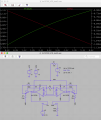I'm quite confused.#### ericgibbs

Joined Jan 29, 2010
15,544
Hi 1919,
Where did you get the LM13700 model.?
I think we should try to determine the model's accuracy and performance.

I will check for any known problems with some of our fellow members.

@Bordodynov @eetech00 @crutschow

E

LTspice LM13700 model problems

#### jacopo1919

Joined Apr 12, 2020
80
the model comes from https://github.com/deanm1278/LM13700-spice-model

***# LM13700-spice-model
Some files for using the lm13700 operational transconductance amplifier (http://www.ti.com/lit/ds/symlink/lm13700.pdf) with LTspice

This model is from here: http://www.idea2ic.com/LM13600/SpiceSubcircuit/LM13700_SpiceModel.html

I have added a VSS terminal for working with split supplies, and have consolidated the default transistor models and the LM13700 subcircuit into one .sub file

I have also created a symbol for LTspice for use in the GUI.***

Within this evening I will try to use the very original TI spice model and run the same simulation.
Thanks a lot for helping out

#### ericgibbs

Joined Jan 29, 2010
15,544
hi 1919,
I would like to see the LTspice results you get from your retesting.
E

#### jacopo1919

Joined Apr 12, 2020
80
Simulation with the TI model (attached), i get :
Vout 4.2V when Ibias is 0.7mA,
Vout 4.38V when Ibias is 1.1mA

If i put them the voltage divider formula (Vin= 5.19V and R2=100k) correspond they to 29k (ibias 0.7mA) and 18.4k (Ibias 1.1 mA).

I can notice is that the measurmentens of this last simulation matches almost precisely the half of what was calculated from post#5
If i put those currents in the formula (Rx= (2 * R)/(gm * RA) , where gm is 19.2 * Ibias):
-for Ibias 0.7mA Rx is 14.8k
-for Ibias 1.1 mA Rx is 9.4k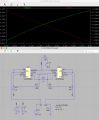#### Attachments

• 1.9 KB Views: 1

#### jacopo1919

Joined Apr 12, 2020
80
In fact, I just noticed from fig. 28 of the datasheet,that Vc is feeding 15V -(-13.6V)/15k=1.9mA split to the 2 Ibias points,

Meaning that maybe TI implies this fact and that Ibias for the calculation in the formual has to be halved.
If this is the case, from your simulation,
0V -(-10.6V)/15k=0.7mA/2=0.35mA
6V -(-10.6V)/15k=1.1mA/2=0.55mA .

If i put those new currents in the formula (Rx= (2 * R)/(gm * RA) , where gm is 19.2 * Ibias):
-for Ibias 0.35mA Rx is 29.76k
-for Ibias 0.55 mA Rx is 18.93k

which are the values that i measured with the original LM13700 TI model

This could be it. mmm
Will need to double check with the single ended VCR version

•ericgibbs

#### ericgibbs

Joined Jan 29, 2010
15,544
Hi 1919,
Checking different simulation idea's I get misleading results.
Check out this method.
E

BTW:
Be aware that Resistors in LTS are polarized, that is, the current flow direction indicated maybe be the reverse of what you expected.
If that happens, use F7 key on the resistor and Ctrl 'R' to rotate the resistor through 180 degs.
Check the 100k current direction in your sim

Updated:
https://electronics.stackexchange.c...rolled-resistor-with-lm13700-how-does-it-work

Clip:

Because in the given circuit the resistor is defined BETWEEN both base nodes of the transistor we have to use the sum of both expressions (Rin1 and Rin2 in series):
Rx=Rin2+Rin1=2(RA+R)/gm*RA.

Up to now, we have not yet considered the fact that the resistor RA is connected not to ground. Instead, it has a common connection to the inverting terminals of BOTH OTAs. Hence it has a "normal" (positive) influence to one OTA (as in the basic model) and a negative influence on the other OTA.
For this reason, the influence of RA will be cancelled out in the sum of both expressions - and we arrive at the given formula: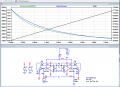#### Attachments

• 3 KB Views: 2
Last edited:

#### jacopo1919

Joined Apr 12, 2020
80
I guess that the nature of the mistake is behind the Spice model since with the one from Github (the one we firstly used and in your last simulation),
Unfortunately I can't add the Rx trace in the Ltspice Plot yet

with Github spice model (below in the picture)
-for Ibias 0.35mA, Vout is 3.79 ---> Rx=36.93k out of the voltage divider formula
-for Ibias 0.55mA, Vout is 3.79 ---> Rx=25.06k out of the voltage divider formula

with the TI spice model (above in the picture)
-for Ibias 0.35mA, Vout is 4.02---> Rx=29.1k out of the voltage divider formula
-for Ibias 0.55mA, Vout is 3.79 ---> Rx=18.49k out of the voltage divider formula

This last one fits matches the formula
Rx= (2 * R)/(gm * RA) , where gm is 19.2 * Ibias:
Rx= (2 * 100000)/(gm * 1000) , where gm is 19.2 * 0.00035A: 29.1k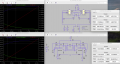#### ericgibbs

Joined Jan 29, 2010
15,544
hi,
Check the indicated current direction in R10 of your original circuit.
E

#### eetech00

Joined Jun 8, 2013
3,162
Hello

I think this is working now. I'm using a modified version of the TI model.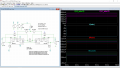Direct Newton iteration for .op point succeeded.
.step vc=0
.step vc=1
.step vc=2
.step vc=3
.step vc=4
.step vc=5
.step vc=6

Measurement: vout
step MAX(v(vout)) FROM TO
1 0 0 0.01
2 0 0 0.01
3 0 0 0.01
4 0 0 0.01
5 0 0 0.01
6 0 0 0.01
7 0 0 0.01

Measurement: iout
step MAX(i(v_iout)) FROM TO
1 0.000159056 0.001 0.003
2 0.00017307 0.001 0.003
3 0.000186937 0.001 0.003
4 0.000200657 0.001 0.003
5 0.000214231 0.001 0.003
6 0.000227659 0.001 0.003
7 0.000240945 0.001 0.003

Measurement: vabc
step MAX(v(abc)) FROM TO
1 -10.598 0.001 0.003
2 -10.5934 0.001 0.003
3 -10.5892 0.001 0.003
4 -10.5853 0.001 0.003
5 -10.5816 0.001 0.003
6 -10.5782 0.001 0.003
7 -10.575 0.001 0.003

Measurement: ibias
step MAX(i(rabc)) FROM TO
1 0.000706535 0.001 0.003
2 0.000772894 0.001 0.003
3 0.000839279 0.001 0.003
4 0.000905684 0.001 0.003
5 0.000972109 0.001 0.003
6 0.00103855 0.001 0.003
7 0.001105 0.001 0.003

Measurement: rx
step (2*100k)/((19.2*(ibias/2))*1k)
1 29486.6
2 26955
3 24822.9
4 23002.9
5 21431.1
6 20060
7 18853.6

Another test circuit.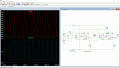•ericgibbs

#### jacopo1919

Joined Apr 12, 2020
80
Hello @eetech00 ! thanks.
Unfortunately is still quite hard for me to understand everything since I just started using LTspice.

I will look further and try to grasp the full meaning but I believe that the text refers at the error log and ''.step param VC 0 6 1'' is a directive that is shows the results of different VC from 0 to 6 V with 1V interval.

From the measurements looks like:

if ibias 0.000706535 = Rx 29.48k
And
ibias 0.001105 = Rx 18.85k

Which is matching the calculations as well as with the original TI spice model.
When you say ''modified'', you mean only on the layout or also the model itself?

@ericgibbs thanks for showing me the polarized nature of resistors on LTpice . I also found this from you here on AAC.
In that case, it was not making any difference if Im not wrong.

•ericgibbs

#### eetech00

Joined Jun 8, 2013
3,162
I will look further and try to grasp the full meaning but I believe that the text refers at the error log and ''.step param VC 0 6 1'' is a directive that is shows the results of different VC from 0 to 6 V with 1V interval.
Yes

From the measurements looks like:

if ibias 0.000706535 = Rx 29.48k
And
ibias 0.001105 = Rx 18.85k
Yes.
I'm using this equation to calculate Rx:

Rx=(2*100k)/((19.2*(ibias/2))*1k)

Notice ibias/2

Which is matching the calculations as well as with the original TI spice model.
When you say ''modified'', you mean only on the layout or also the model itself?
I modified the TI spice model. The original had some .trans startup issues and wouldn't work for the Tri-to-Square circuit.

•jacopo1919